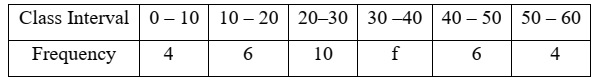ICSE Class 10 Mathematics 2022 Semester-2 Sample Paper

Class 10 Maths

Maximum marks are 40. Time allowed is one and a half hours. You have to attempt all questions from Section A and any three questions from Section B.

Section A

Question 1

Choose the correct answers to the questions from the given options. 

(i) The point (3,0) is invariant under reflection in:

(a) The origin
(b) x-axis
(c) y-axis
(d) both x and y axes

(ii) In the given figure, AB is a diameter of the circle with centre O. If ∠COB = 55⁰ then the value of x is: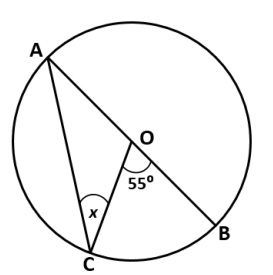(a) 27.5⁰
(b) 55⁰
(c) 110⁰
(d) 125⁰

(iii) If a rectangular sheet having dimensions 22 cm x 11 cm is rolled along its shorter side to form a cylinder. Then the curved surface area of the cylinder so formed is:

(a) 968 cm2
(b) 424 cm2
(c) 121 cm2
(d) 242 cm2

(iv) If the vertices of a triangle are (1,3), (2, - 4) and (-3, 1). Then the co-ordinate of its centroid is:

(a) (0, 0)
(b) (0, 1)
(c) (1, 0)
(d) (1, 1)

(v) tan θ x √(1 - sin2 θ) is equal to:

(a) cos θ
(b) sin θ
(c) tan θ
(d) cot θ

(vi) The median class for the given distribution is: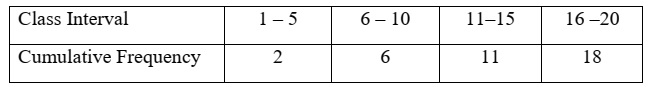(a) 1 - 5
(b) 6 - 10
(c) 11 - 15
(d) 11 - 20

(vii) If the lines 7y = ax + 4 and 2y = 3 - x are parallel to each other, then the value of a is:

(a) -1
(b) -7/2
(c) -2/7
(d) 14

(viii) Volume of a cylinder is 330 cm3. The volume of the cone having same radius and height as that of the given cylinder is:

(a) 330 cm3
(b) 165 cm3
(c) 110 cm3
(d) 220 cm3

(ix) In the given graph, the modal class is the class with frequency: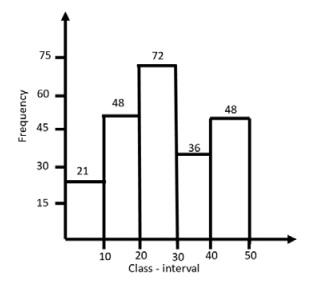(a) 72
(b) 21
(c) 48
(d) 36

(x) If the probability of a player winning a game is 0.56. The probability of his losing this game is:

(a) 0.56
(b) 1
(c) 0.44
(d) 0

Section B

Question 2

(i) Find the ratio in which the x-axis divides internally the line joining points A(6, -4) and B(-3, 8). 

(ii) Three rotten apples are accidently mixed with twelve good ones. One apple is picked at random. What is the probability that it is a good one? 

(iii) In the given figure, AC is a tangent to circle at point B. ∆EFD is an equilateral triangle and ∠CBD = 40°.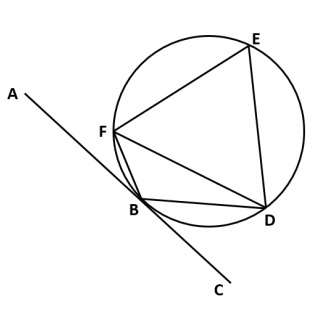Find: 

1. ∠BFD
2. ∠FBD
3. ∠ABF

(iv) A drone camera is used to shoot an object P from two different positions R and S along the same vertical line QRS. The angle of depression of the object P from these two positions are 35° and 60° respectively as shown in the diagram. If the distance of the object P from point Q is 50 metres, then find the distance between R and S correct to the nearest meter.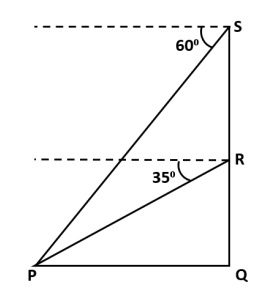Question 3

(i) In the given figure, PT is a tangent to the circle at T, chord BA is produced to meet the tangent at P. Perpendicular BC bisects the chord TA at C. If PA = 9 cm and TB = 7 cm, find the lengths of: 

1. AB
2. PT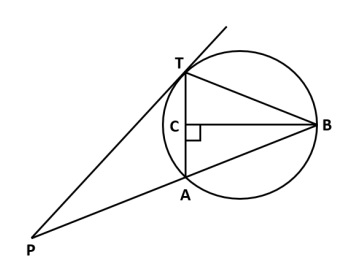(ii) How many solid right circular cylinders of radius 2 cm and height 3 cm can be made by melting a solid right circular cylinder of diameter 12 cm and height 15 cm? 

(iii) Prove that: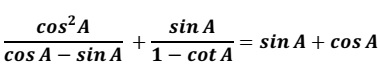(iv) Use graph paper for this question, take 2 cm = 10 marks along one axis and 2 cm = 10 students along the other axis.

The following table shows the distribution of marks in a 50 marks test in Mathematics: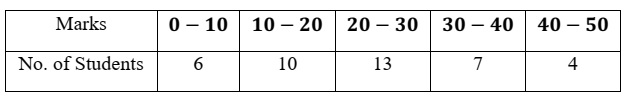Draw the ogive for the above distribution and hence estimate the median marks. 

Question 4

(i) Find the equation of the perpendicular dropped from the point P(-1,2) onto the line joining A(1,4) and B(2,3). 

(ii) Find the mean for the following distribution: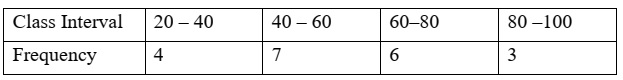(iii) A solid piece of wooden cone is of radius OP = 7 cm and height OQ = 12 cm. A cylinder whose radius and height equal to half of that of the cone is drilled out from this piece of wooden cone. Find the volume of the remaining piece of wood.(Use π = 22/7)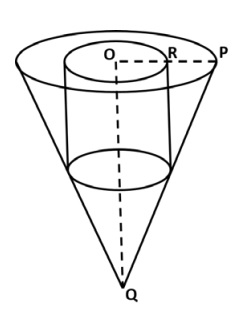(iv) Use a graph sheet for this question, take 2 cm = 1 unit along both x and y axis: 

1. Plot the points A(3, 2) and B(5, 0). Reflect point A on the y-axis to A΄. Write co-ordinates of A΄.
2. Reflect point B on the line AA΄ to B΄. Write the co-ordinates of B΄.
3. Name the closed figure A’B’AB.

Question 5

(i) In the given figure, the sides of the quadrilateral PQRS touches the circle at A, B, C and D. If RC = 4 cm, RQ = 7 cm and PD = 5 cm. Find the length of PQ: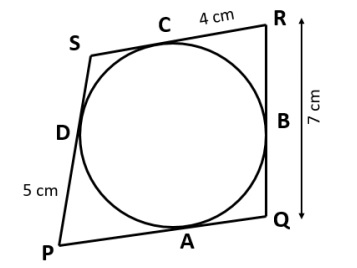(ii) Prove that: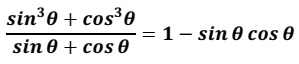(iii) In the given diagram, OA = OB, ∠OAB = θ and the line AB passes through point P(-3, 4).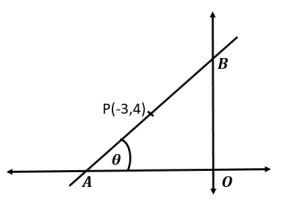Find:

1. Slope and inclination (θ) of the line AB
2. Equation of the line AB

(iv) Use graph paper for this question. Estimate the mode of the given distribution by plotting a histogram. [Take 2 cm = 10 marks along one axis and 2 cm = 5 students along the other axis]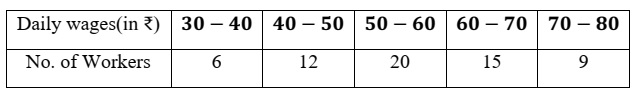Question 6

(i) A box contains tokens numbered 5 to 16. A token is drawn at random. Find the probability that the token drawn bears a number divisible by: 

1. 5
2. Neither by 2 nor by 3

(ii) Point M(2, b) is the mid-point of the line segment joining points P(a, 7) and Q(6, 5). Find the values of a and b. 

(iii) An aeroplane is flying horizontally along a straight line at a height of 3000 m from the ground at a speed of 160 m/s. Find the time it would take for the angle of elevation of the plane as seen from a particular point on the ground to change from 60⁰ to 45⁰. Give your answer correct to the nearest second. 

(iv) Given that the mean of the following frequency distribution is 30, find the missing frequency f